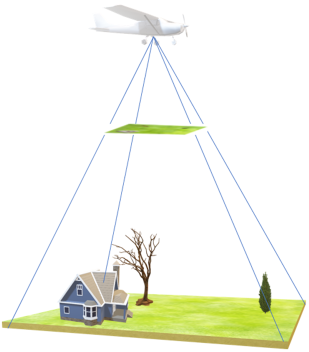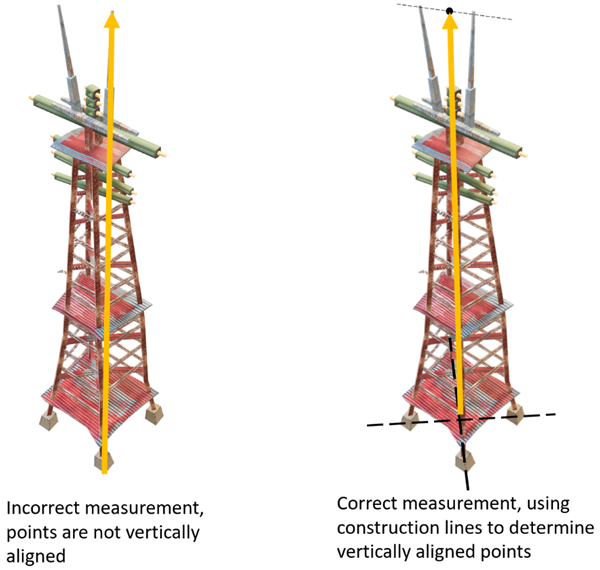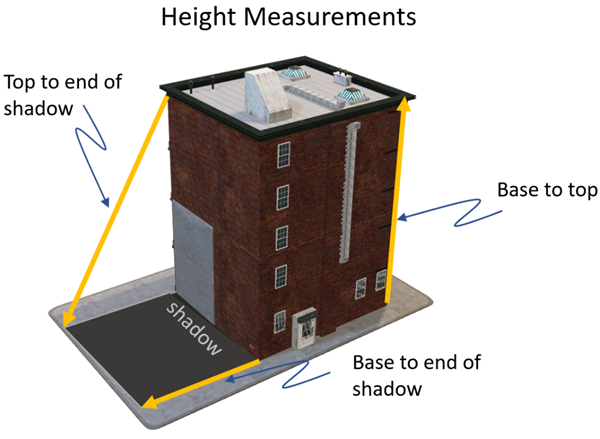# Mensuration

## Resumen

Performs measurements on an image with a sensor model, which includes the geometric sensor model and the appropriate metadata (Image Support Data) that is supplied with the image. The supported types of measurements include point, distance or length, area, and feature height.

## Debate

Image mensuration relies on the image-to-ground transformation of a sensor model. For single image mensuration, the transformations require the elevation of the point. The height can be estimated with an average height, but a terrain model will provide more accurate results. The mensuration computation requires image x,y coordinates and a ground z-coordinate. When a terrain model is included, the calculation is iterative, intersecting a ray from the image to the terrain model to determine the point intersection with the ground. In rare instances, particularly in the cases of rugged terrain and high obliquity, the iteration may not result in a valid solution because of ambiguity. In these cases, the system is unable to compute a singular point, resulting in no valid solution.

##### Nota:

It is possible for the measurement methods (point, length, area, and feature height) to not return a value if a solution is indeterminate. It is important to only use image coordinates with this function since map coordinates will first be transformed to image space, resulting in possible error.Calculate the center point of an image.

``````import arcpy
raster = arcpy.sa.Raster(raster_path)
mens = arcpy.ia.Mensuration(raster)
result = mens.point((raster.width/2, raster.height/2))
print(f"Center: ({result.lat}, {result.lon})")``````

For methods where two points are used, a distance and direction will be returned. Distance is a linear measurement along the ground plane. The direction is the azimuth from north of the line defined from the first measured point to the second measured point. It is important to measure points in the image that are along flat terrain.

For height measured from the base of an object to the top of the object, it is assumed that the points are vertically aligned, meaning that the lower point is directly below the upper point. For measurements using shadows, sun angle information must be included in the sensor model metadata. It is assumed that an object casts a shadow onto flat terrain that intersects the base of the object at a point that is understood to be the base. If the terrain is sloped, it can either extend or shorten the returned height value.Calculate the distance along the top of an image.

``````import arcpy
raster = arcpy.sa.Raster(raster_path)
mens = arcpy.ia.Mensuration(raster)
result = mens.distance((0, 0), (raster.width, 0))
print(f"Image Width: {result.distance} m")``````

The area calculation uses the image’s sensor model and defined terrain to calculate the ground coverage and perimeter on the image. This is computed on the ellipsoid and does not follow the terrain. It is possible for this method to not return a value if it is unable to converge on a solution. It is important to only use image coordinates with this function, as map coordinates will first be transformed to image space, resulting in a possible error.

Calculate the ground area of an image.

``````import arcpy
raster = arcpy.sa.Raster(raster_path)
mens = arcpy.ia.Mensuration(raster)
result = mens.area([(0, 0), (raster.width, 0),
(raster.width, raster.height), (0, raster.height)])
print(f"Image Size: {result.area} m^2")``````

The height calculation is computed on the ellipsoid and does not follow the terrain. It is possible that this method will not return a value if it is unable to converge on a solution. It is important to only use image coordinates (column, row) with this method, as map coordinates will be transformed to image space, resulting in a possible error.Calculate the height of an object:

``````import arcpy
raster = arcpy.sa.Raster(raster_path)
mens = arcpy.ia.Mensuration(raster)
result = mens.height((base_x, base_y),
(top_x, top_y),
Mensuration.base_to_top)
print (f"Height: {result.height} m")``````

## Sintaxis

```Mensuration
(raster)```
 Parámetro Explicación Tipo de datos raster A raster object created with the Raster class, or the path to a raster dataset. Raster

 Propiedad Explicación Tipo de datos base_to_shadow(Lectura y escritura) Calculates the height of a feature by measuring from the base of the object to the top of the object's shadow on the ground. Long base_to_top(Lectura y escritura) Calculates the height of a ground feature by measuring from the base of the object to the top of the object. Long top_to_shadow(Lectura y escritura) Calculates the height of a feature by measuring from the top of the object to the top of the object's shadow on the ground. Long

## Descripción general del método

 Método Explicación area (polygon) Calculates the area of a polygon in an image, based on a sensor model. distance (from_point, to_point) Calculates the geographic distance between two points in an image, based on a sensor model. height (base_point, top_point, height_type) Calculate the vertical height of an object. point (point) Calculates the geographic coordinate based on sensor model.

## Métodos

`area (polygon)`
 Parámetro Explicación Tipo de datos polygon The input polygon. The polygon can be specified as a Polygon object, a list of Point objects, or a list of coordinate pairs in tuples.If specified as a list of tuples, the x- and y-coordinate values must be in the image coordinate system (column, row). If other spatial reference systems are preferred, use Polygon or Point objects with a spatial reference system defined. Polygon
Valor de retorno
 Tipo de datos Explicación AreaMeasurement The computed area values. The following attributes are returned:area (float)—The area in square meters. perimeter (float)—The perimeter in meters.sensor_name (string)—The sensor model name.

Calculate the ground area of an image.

``````import arcpy
in_raster = arcpy.sa.Raster('Charlotte_multiband.tif')
mensuration = arcpy.ia.Mensuration(in_raster)
result = mensuration.area([(0, 0), (in_raster.width, 0), (in_raster.width, in_raster.height), (0, in_raster.height)])
print(f"Image Size: {result.area} m^2")``````
`distance (from_point, to_point)`
 Parámetro Explicación Tipo de datos from_point The input coordinates of the origin point. The point can be specified as a Point object, a PointGeometry object, or a coordinate pair in a tuple.If specified as a tuple, the x- and y-coordinate values must be in an image coordinate system (column, row). If other spatial reference systems are preferred, use the Point or PointGeometry objects with a spatial reference system defined. Point to_point The input coordinates of the destination point. The point can be specified as a Point object, a PointGeometry object, or a coordinate pair in a tuple.If specified as a tuple, the x-and y-coordinate values must be in the image coordinate system (column, row). If other spatial reference systems are preferred, use Point or PointGeometry objects with a spatial reference system defined. Point
Valor de retorno
 Tipo de datos Explicación LinearMeasurement The computed distance values. The following attributes are returned: distance (float)—The distance in meters. azimuth (float)—The azimuth angle in decimal degrees.sensor_name (string)—The sensor model name.

Calculate the distance along the top of an image.

``````import arcpy
in_raster = arcpy.Raster('Charlotte_multiband.tif')
mensuration = arcpy.ia.Mensuration(in_raster)
result = mensuration.distance((0, 0),( raster.width, 0))
print(f"Image Width: {result.distance} m")``````
`height (base_point, top_point, height_type)`
 Parámetro Explicación Tipo de datos base_point The input coordinates of the base of the feature to measure. This can be specified as a Point object, a PointGeometry object, or a coordinate pair in a tuple.If specified as a tuple, the x- and y-coordinate values should be in the image coordinate system (column, row). If other spatial reference systems are preferred, use PointGeometry or Point objects with a spatial reference system defined. Point top_point The input coordinates of the top of the feature to measure. The point can be specified as a Point object, a PointGeometry object, or a coordinate pair in a tuple.If specified as a tuple, the x- and y-coordinate values should be in the image coordinate system (column, row). If other spatial reference systems are preferred, use PointGeometry or Point objects with a spatial reference system defined. Point height_type Height measurements of an object can be obtained when a sensor model exists for an image. Sun angle information is required to make measurements using shadows. The height of an object is calculated between two points, based on a sensor model. Three types of height measurements are supported:base_to_top—Calculates the height of a ground feature by measuring from the base of the object to the top of the object.base_to_shadow—Calculates the height of a feature by measuring from the base of the object to the top of the object's shadow on the ground.top_to_shadow—Calculates the height of a feature by measuring from the top of the object to the top of the object's shadow on the ground. Integer
Valor de retorno
 Tipo de datos Explicación HeightMeasurement The computed height values. The following attributes are returned: height (float)—The height in meters.sensor_name (string)—The sensor model name.

Calculate the height of an object.

``````import arcpy
in_raster = arcpy.sa.Raster('Charlotte_multiband.tif')
mensuration = arcpy.ia.Mensuration(in_raster)
result = mensuration.height((base_x, base_y), (top_x, top_y), Mensuration.base_to_top)
print(f"Height: {result.height} m")``````
`point (point)`
 Parámetro Explicación Tipo de datos point The input coordinates to measure. The point can be specified as a Point object, a PointGeometry object, or a coordinate pair in a tuple.If specified as a tuple, the x- and y-coordinate values must be in the image coordinate system (column, row). If other spatial reference systems are preferred, use Point or PointGeometry objects with a spatial reference system defined. Point
Valor de retorno
 Tipo de datos Explicación PointMeasurement The computed ground location. The following attributes are returned:lon (float)—The longitude in decimal degrees (WGS84).lat (float)—The latitude in decimal degrees (WGS84).elev (float)—The elevation in meters (HAE).sensor_name (string)—The sensor model name.

Return the latitude and longitude values of the center point of an image.

``````import arcpy
in_raster = arcpy.Raster('Charlotte_multiband.tif')
mensuration = arcpy.ia.Mensuration(in_raster)
result = mensuration.point((in_raster.width/2, in_raster.height/2))
print(f"Center: ({result.lat}, {result.lon})")``````